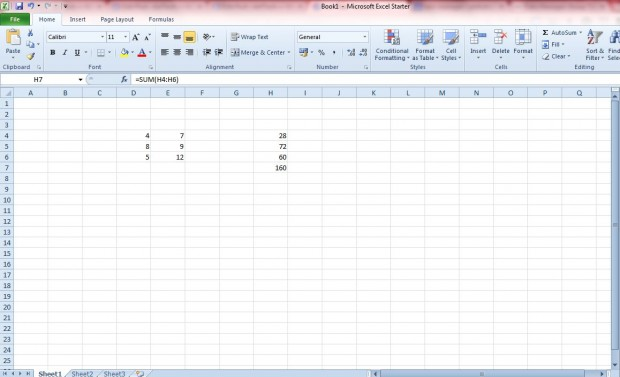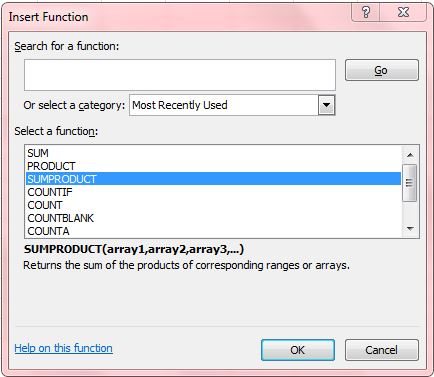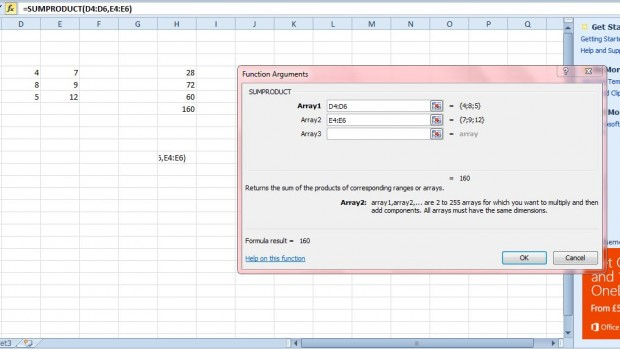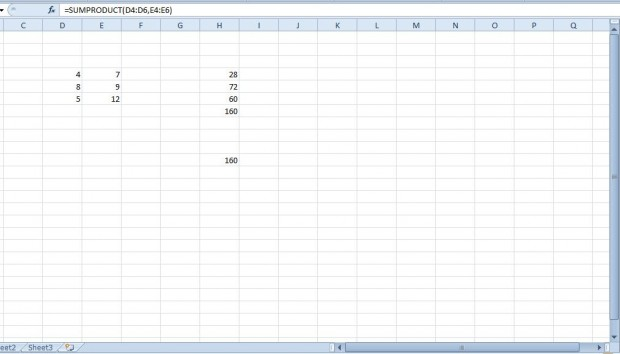# How to use SUMPRODUCT formula in Excel [Tip]

Excel is one of the best spreadsheet applications that has a multitude of functions to select from. Among those functions is SUMPRODUCT. That’s a function that takes one or more arrays of numbers and adds the sum of their products together.

For example, enter four, seven, eight, nine, five and 12 into two columns of six Excel spreadsheet cells as shown in the shot directly below. To find the sum of their products, you could first multiply four x seven, eight x nine and five x 12 with the PRODUCT function. That would give you 28, 72 and 60. Then add those together with the SUM function in Excel, which would give you 160.However, SUMPRODUCT wraps both the multiplication and summing of those cells into one function. Click on a cell, and then press the Insert Function (fx) button to open the window in the shot below. Then select SUMPRODUCT from that window.That will open the window below. There select one column for Array1 and one for Array2. In our example, they would be the column that includes four, eight and five and the second column with seven, nine and 12 in its cells.Then press the OK button on that window. The function will then add the products of each of the rows in the two columns to give you a total of 160 as shown below.So with this function you can quickly sum product values together. That’s much better than setting up two separate functions that will first multiply two, or more, numbers across various rows and then sum the totals together. You can add the function to both Excel 2010/2013 spreadsheets, but some of the earlier versions might not include it.